# MCQ Questions for Class 12 Chemistry Chapter 12 Aldehydes, Ketones and Carboxylic Acids with Answers

Students are advised to practice the NCERT MCQ Questions for Class 12 Chemistry Chapter 12 Aldehydes, Ketones and Carboxylic Acids with Answers Pdf free download is available here. MCQ Questions for Class 12 Chemistry with Answers are prepared as per the Latest Exam Pattern. Students can solve these Aldehydes, Ketones and Carboxylic Acids Class 12 MCQs Questions with Answers and assess their preparation level.

## Aldehydes, Ketones and Carboxylic Acids Class 12 MCQs Questions with Answers

Solving the Aldehydes, Ketones and Carboxylic Acids Multiple Choice Questions of Class 12 Chemistry Chapter 12 MCQ can be of extreme help as you will be aware of all the concepts. These MCQ Questions on Aldehydes, Ketones and Carboxylic Acids Class 12 with answers pave for a quick revision of the Chapter thereby helping you to enhance subject knowledge. Have a glance at the MCQ of Chapter 12 Chemistry Class 12 and cross-check your answers during preparation.

Question 1.
Addition of water to alkynes occurs in acidic medium and in the presence of Hg2+ ions as a catalyst. Which of the following products will be formed on addition of water to but-1 -yne under these conditions?Answer

Answer: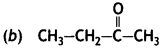Question 2.
Which of the following compounds is most reactive towards nucleophilic addition reactions?Answer

Answer: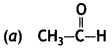Question 3.
The correct order of increasing acidic strength is
(a) Phenol < Ethanol < Chloroacetic acid < Acetic acid
(b) Ethanol < Phenol < Chloroacetic acid < Acetic acid
(c) Ethanol < Phenol < Acetic acid < Chloroacetic acid
(d) Chloroacetic acid < Acetic acid < Phenol < Ethanol

Answer

Answer: (c) Ethanol < Phenol < Acetic acid < Chloroacetic acid

Question 4.
Compound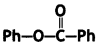can be prepared by the reaction of
(a) Phenol and benzoic acid in the presence of NaOH
(b) Phenol and benzoyl chloride in the presence of pyridine
(c) Phenol and benzoyl chloride in the presence of ZnCl2
(d) Phenol and benzaldehyde in the presence of palladium

Answer

Answer: (b) Phenol and benzoyl chloride in the presence of pyridine

Question 5.
The reagent which does not react with both, acetone and benzaldehyde.
(a) Sodium hydrogensulphite
(b) Phenyl hydrazine
(c) Fehling’s solution
(d) Grignard reagent

Answer

Answer: (c) Fehling’s solution

Question 6.
Cannizzaro’s reaction is not given by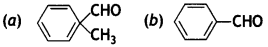(c) HCHO
(d) CH3CHO

Answer

Answer: (d) CH3CHO

Question 7.
Which product is formed when the compoundis treated with concentrated aqueous KOH solution?Answer

Answer:Question 8.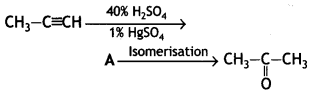Structure of ‘A’ and type of isomerism in the above reaction are respectively.
(a) Prop-1-en-2-ol, metamerism
(b) Prop-1 -en-1 -ol, tautomerism
(c) Prop-2-en-2-ol, geometrical isomerism
(d) Prop-1-en-2-ol, tautomerism

Answer

Answer: (d) Prop-1-en-2-ol, tautomerism

Question 9.
Compounds A and C in the following reaction are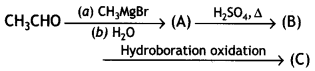(a) identical
(b) positional isomers
(c) functional isomers
(d) optical isomers

Answer

Answer: (b) positional isomers

Question 10.
Which is the most suitable reagent for the following conversion?(a) Tollen’s reagent
(b) Benzoyl peroxide
(c) l2 and NaOH solution
(d) Sn and NaOH solution

Answer

Answer: (c) l2 and NaOH solution

Question 11.
Which of the following compounds wilt give butanone on oxidation with alkaline KMnO4 solution?
(a) Butan-1-ol
(b) Butan-2-ol
(c) Both of these
(d) None of these

Answer

Answer: (b) Butan-2-ol

Question 12.
In Clemmensen Reduction carbonyl compound is treated with
(a) Zinc amalgam + HCl
(b) Sodium amalgam + HCl
(c) Zinc amalgam + nitric add
(d) Sodium amalgam + HNO3

Answer

Answer: (a) Zinc amalgam + HCl

Assertion and Reason Type Questions

The questions given below consist of an assertion and a reason. Use the following key to choose the appropriate answer.
(a) Both Assertion (A) and Reason (R) are correct statements, and Reason (R) is the correct explanation of the Assertion (A).
(b) Both Assertion (A) and Reason (R) are correct statements, but Reason (R) is not the correct explanation of the Assertion (A).
(c) Assertion (A) is correct, Reason (R) is wrong statement.
(d) Assertion (A) is wrong, but Reason (R) is correct statement.

Question 13.
Assertion: Formaldehyde is a planar molecule.
Reason: It contains sp² hybridised carbon atom.

Answer

Answer: (a) Both Assertion (A) and Reason (R) are correct statements, and Reason (R) is the correct explanation of the Assertion (A).

Question 14.
Assertion: Compounds containing -CHO group are easily oxidised to corresponding carboxylic acids.
Reason: Carboxylic acids can be reduced to alcohols by treatment with LiAlH4.

Answer

Answer: (b) Both Assertion (A) and Reason (R) are correct statements, but Reason (R) is not the correct explanation of the Assertion (A).

Question 15.
Assertion: The a-hydrogen atom in carbonyl compounds is less acidic.
Reason: The anion formed after the loss of α-hydrogen atom is resonance stabilised.

Answer

Answer: (d) Assertion (A) is wrong, but Reason (R) is correct statement.

Question 16.
Assertion: Aromatic aldehydes and formaldehyde undergo Cannizzaro reaction.
Reason: Aromatic aldehydes are almost as reactive as formaldehyde.

Answer

Answer: (c) Assertion (A) is correct, Reason (R) is wrong statement.

Question 17.
Assertion: Aldehydes and ketones, both react with Tollen’s reagent to form silver mirror.
Reason: Both, aldehydes and ketones contain a carbonyl group.

Answer

Answer: (d) Assertion (A) is wrong, but Reason (R) is correct statement.

Hope the information shared regarding the NCERT MCQ Questions for Class 12 Chemistry Chapter 12 Aldehydes, Ketones and Carboxylic Acids with Answers Pdf free download is true and genuine as far as our knowledge is concerned. If you feel any information regarding CBSE Class 12 Chemistry Aldehydes, Ketones and Carboxylic Acids MCQs Multiple Choice Questions with Answers is missing do reach us and we will look into it and add it accordingly.

error: Content is protected !!